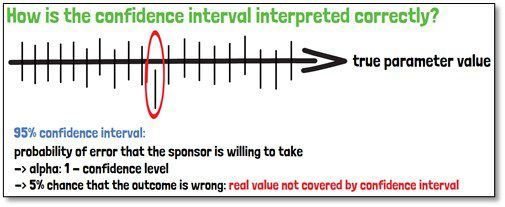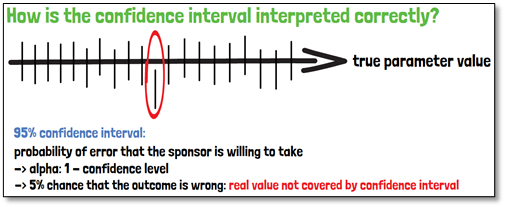# What is a confidence interval?Investigators in clinical trials are interested in whether or not the treatment effect of a new product or device is clinically significant. Frequently statistical outcomes are leaned on p-values, which in fact are only showing weather the effect is statistically significant or not. The appropriate tool here is the confidence interval. It is used to interpret the precision of the estimate. In contrast to statistical tests,
confidence intervals also estimate in which interval the estimates range. They can be calculated for all parameters that are estimated from data, e.g., effect sizes, parameters, correlation coefficients. It is a range of confidence with a certain predefined error probability, a measure of precision of the estimate.

The calculation of the confidence interval is done through the point estimator, the standard error, as well as a quantile of the distribution of the point estimator. Before a confidence interval is calculated, the confidence level must be determined. It is the proportion of intervals, that contain the true population parameter of interest, in a set of repeated sampling. It is commonly chosen to be 95%, but also 90 or 99%.

The width of the confidence interval is what defines its precision. Therefore, the interval is desired to be as narrow as possible, as this indicates a precise estimate of the true value. The width of a confidence interval is influenced by three parameters:

1. The larger the sample size is, the smaller is the width of the confidence interval, and the more precise it will be.
2. The smaller the standard error, the smaller will be also the width of the confidence interval.
3. Finally, the confidence level has an impact. The lower the confidence level is, the smaller will be the width of the confidence interval. Since the larger is the confidence, the less precise will be the interval.

The Confidence interval on a 95% level is the interval that covers in average the true parameter value in 95 of 100 repeated random samples taken from the same population.The confidence level is reflecting the probability of error that the sponsor is willing to take in return, also called alpha. In case of the 95% confidence interval, that would mean, there is a 5% chance that the outcome is wrong, and the real value is not covered by the interval.

If you are interested in a visual presentation of this topic, please feel free to follow us on our GCP Mindset YouTube channel! If you would like to know more about how we could implement statistics in your clinical trial, send us a mail to statistics@gcp-service.com.